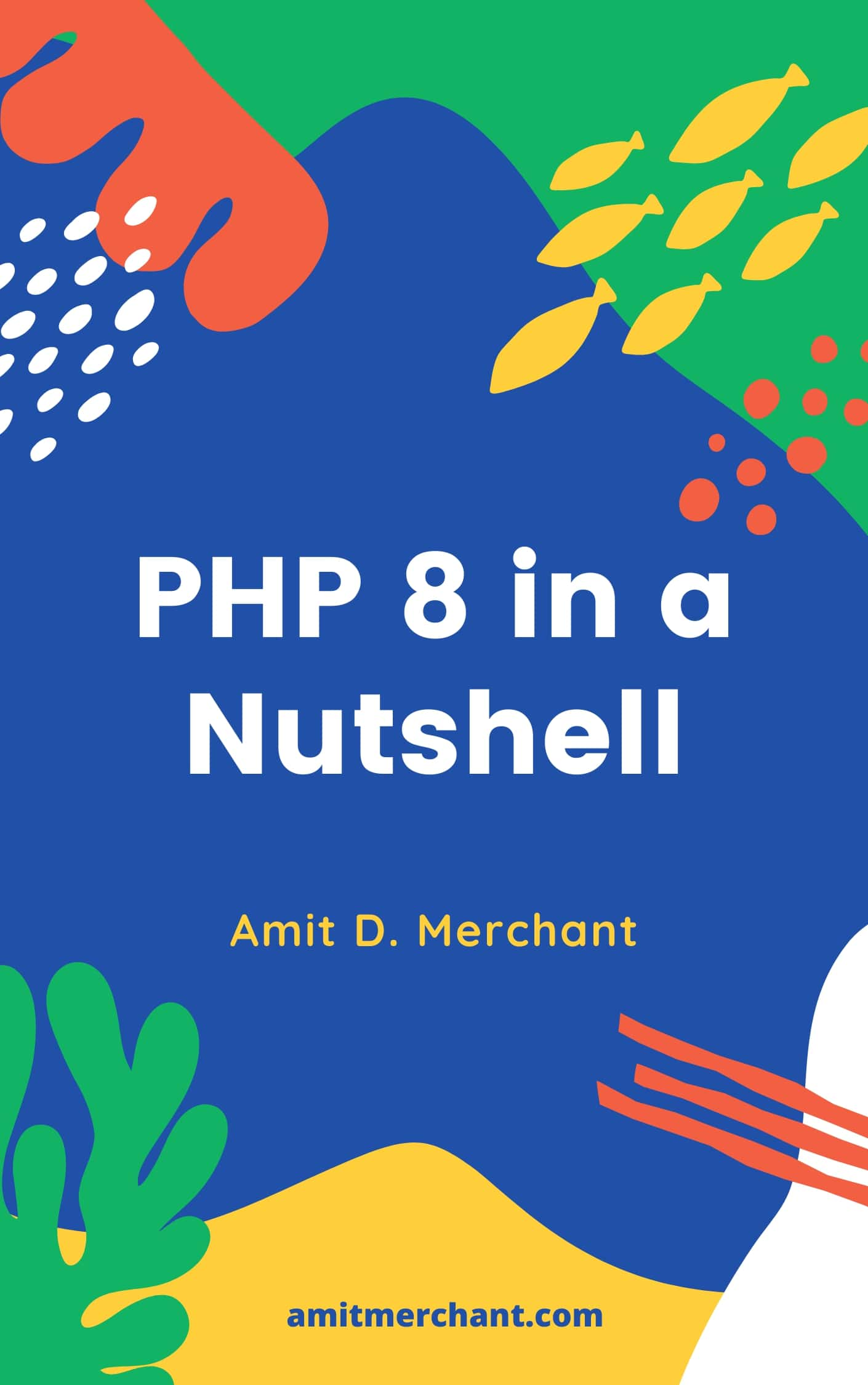# Amit MerchantVerified (\$10/year for the domain)

A blog on PHP, JavaScript, and more

# Using arrow functions in PHP 7.4

When PHP 7.4 released, it came with a whole lot of features/improvements that makes the language more interesting to work with. The one such feature that I want to talk about is arrow functions. For a primer, arrow functions are not new. In fact, If you’ve been working with the latest JavaScript (EcmaScript 6), you might’ve worked with arrow functions already.

## Before PHP 7.4

With PHP 7.4, you can now use a shorthand syntax for defining functions with implicit by-value scope binding. Let’s understand it by taking the following example.

``````<?php

\$factor = 10;

\$numbers = array_map(function(\$value) use (\$factor){
return \$value * \$factor;
}, [1, 2, 3]);

print_r(\$numbers);
//Array( => 10  => 20  => 30)

?>
``````

As you can see, in the above example, if you want to use higher order functions such as array_map, there will be an anonymous function which will evaluate the values from the array passed as a second argument and if you want to use a variable which lies outside of the scope of the anonymous function, you’ll need to add `use`. That’s a lot of boilerplate code, right?

## Hello, Arrow functions!

From PHP 7.4, the above can be reduced to the following using fat arrow(`=>`) syntax like so.

``````<?php

\$factor = 10;

\$numbers = array_map(fn(\$value) => \$value * \$factor, [1, 2, 3]);

print_r(\$numbers);
//Array( => 10  => 20  => 30)

?>
``````

The entire code is now reduced to just one line. It’s more readable, shorter and cleaner now. And as an added bonus, you now don’t have to pass the local varible (in above example `\$factor`) using `use` explicitly. It’ll be accessible in the arrow function automatically.

Also, because arrow functions have an implicit “return”, it makes them ideal for single expressions rather than blocks of procedural code.

## The limitation

There’s one limitation however using arrow functions. i.e. Currently, PHP only supports arrow functions for anonymous functions which consists of only one line. So, something like following is not supported yet.

``````<?php

\$factor = 10;

\$numbers = array_map(fn(\$value) => {
\$factor = \$factor * 2;

return \$value * \$factor;
}, [1, 2, 3]);

print_r(\$numbers);
//Array( => 10  => 20  => 30)

?>
``````

Hopefully, this will get fixed in the next iterations of PHP.

Fingers crossed!

👋 Hi there! I'm Amit. I write articles about all things web development. If you like what I do and want me to continue doing the same, I'd like you consider leaving a tip. I'd highly appreciate that. Cheers!(Includes PHP 8.1 and 8.2)

My Tiny YouTube Channel ➔